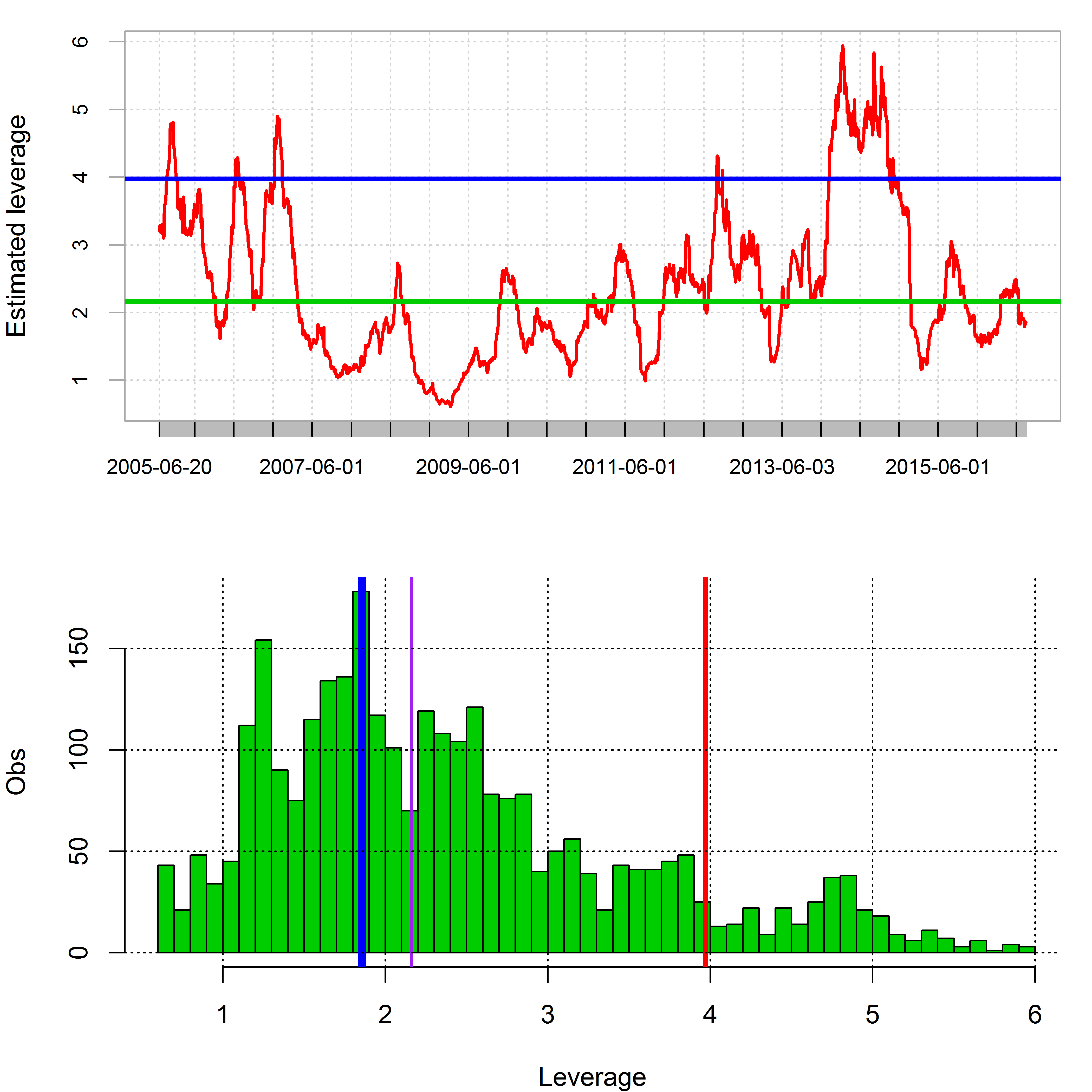# G10 FX Position Report Update

G10 FX POSITIONING REPORT

Sun Jul 17 21:02:23 2016

The following report aims to provide a gauge to the current market positioning in G10 FX. It focuses on US\$ crosses and uses a standardised statistical measures of price deviation as well as a regression methodology to produce an estimate of how stretched currency exchange rates are and also to evaluate how currency managers are likely to be positioned and leveraged in G10 Currency. I use the BTOPFX in the report but can do the computations for any other peer group benchmark.

G10 FX STRETCH MAP

The stretch indicator looks at how much exchange rates are extended by calculating the T-stat of the mean price deviation over a rolling period of 61 days. The charts below shows the results for each currency pairs over the last 500 days. The spot prices are expressed as 1 unit of foreign currency versus the USD. The purple line represent the median value since 2005 and the red lines represent the 95% confidence intervals. Therefore if the value is above or below those the deviation of the given exchange rate would be deemed as atypical relative to what would be expected under a normal distribution and therefore overbought/oversold.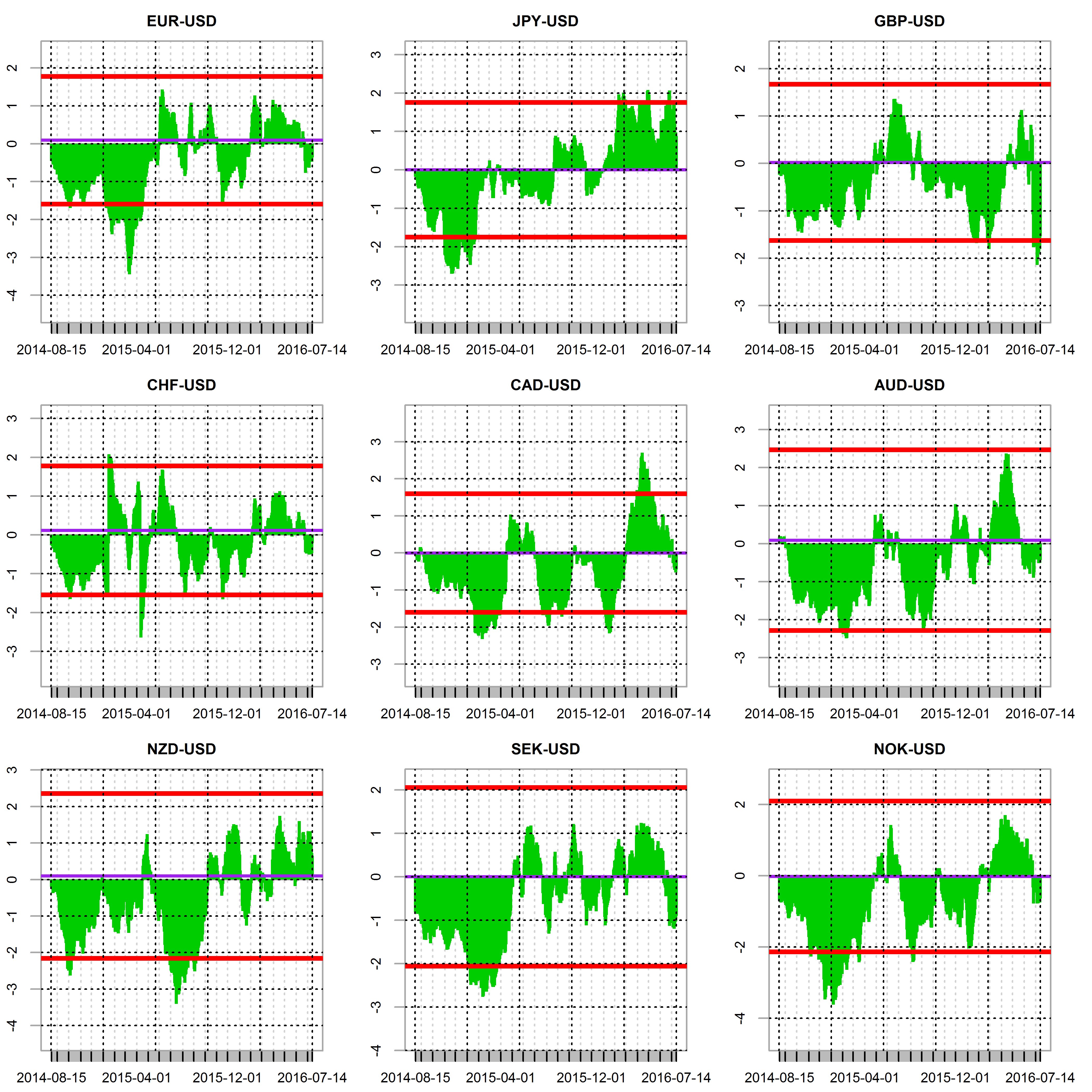The below shows the above calculated T-stats but this time relative to their historical distributions. Once again the red lines delimit the 95% confidence intervals and the purple line the median value. The blue line indicates the most current value of the T-stat.The following Map chart shows how stretched G10 FX exchange rates are over time horizons ranging from 1-month to 6-month. The bigger the square the most significant the upside (green) or downside (red) of the exchange rate over the given period. All the exchange rates are quoted on CCY-US\$ basis so red indicate a depreciation of a given CCY against US\$ and green an appreciation versus the US\$.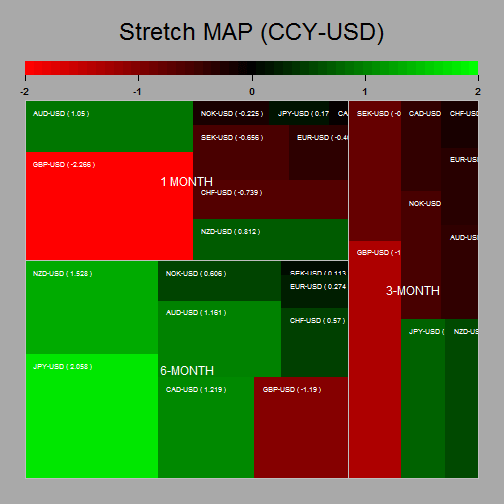Estimated Currency Managers Positioning in G10 FX

To determine the sensitivity of currency managers to exchange rates and therefore their current positioning we regress the daily returns of the BTOPFX index against the daily logarithmic returns of G10 FX rates. We then calculate the T-stat for each of the regression’s slope coefficients. The higher the T-stat the higher the sensitivity to a given currency and therefore likely positioning. Using the regression weights as well as the variance of the independent and explanatory variables as input we can then easily deduce an estimation of the current risk utilisation of the typical currency manager as inferred by the values of the BTOPFX.

The below shows the T-stat of the regression’s slope coefficients over the last 500 days. The purple line represents the median value since 2005 and the red lines represent the 95% confidence intervals. Therefore if the value is above or below the red lines the positioning in a currency would be deemed as extreme and therefore the risk of unwinding would be greater since the market inventory would likely be close to its highest. Probably highlighting a good environment to enter a contrarian trade.The sensitivity of currency managers returns to changes in G10 FX rates relative to their historical distribution is shown below. Once again the red lines are the 95% confidence intervals and the purple line the median value. The blue line indicates the most current value of the T-stat. If this one is either side of the intervals of confidence it indicates a potentially overextended market positioning in the given currency.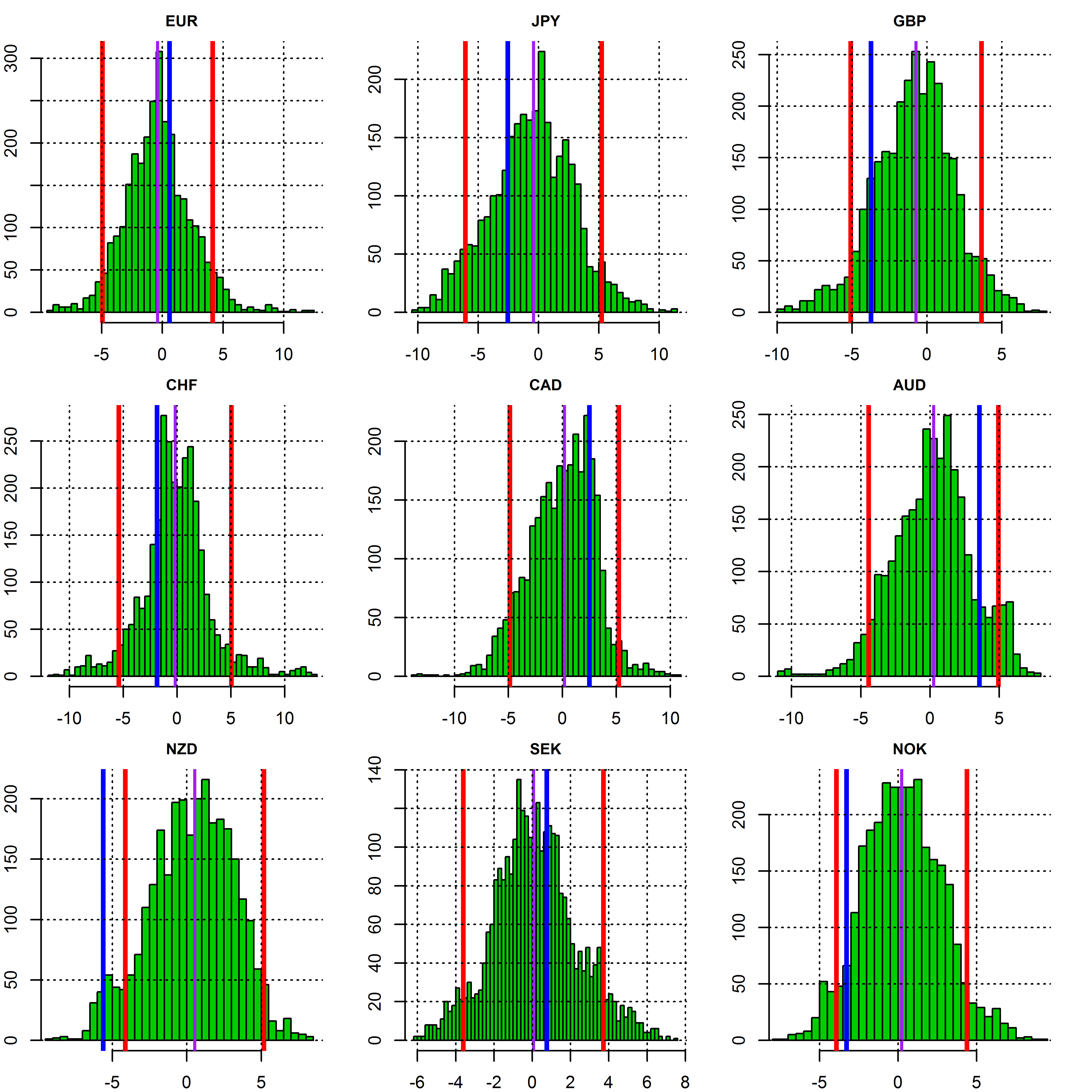The exposure to the US\$ is derived from the combined sensitivities to the other currencies and is shown in the same fashion than for the other currencies. Namely against an axis of time and relative to its historical distribution.The below Map chart shows the currency managers sensitivity to G10 FX exchange rates over time horizons ranging from 1-month to 6-month. The bigger the square the most significant the sensitivity to a currency the exchange rate over the given period. Long positioning is shown in green and short in red.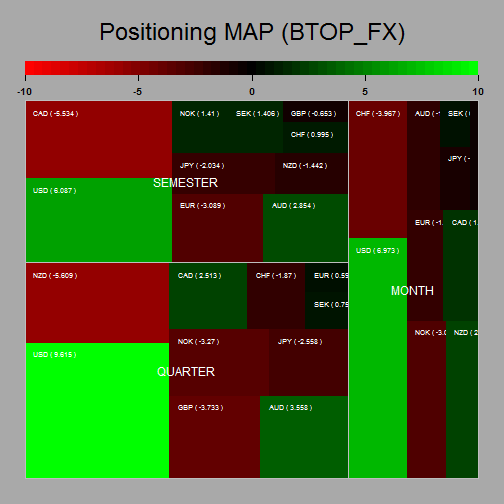Estimated Leverage

As explained previously the level of risk utilisation of currency managers and therefore their gearing can easily be derived by using the regression coefficients and the variances of both the independent and explanatory variables. The chart below shows the rolling estimation of risk utilisation as well putting it in respect of its historical distribution. Average Risk utilisation over the last 61 days is estimated at 31.24 % of maximum.